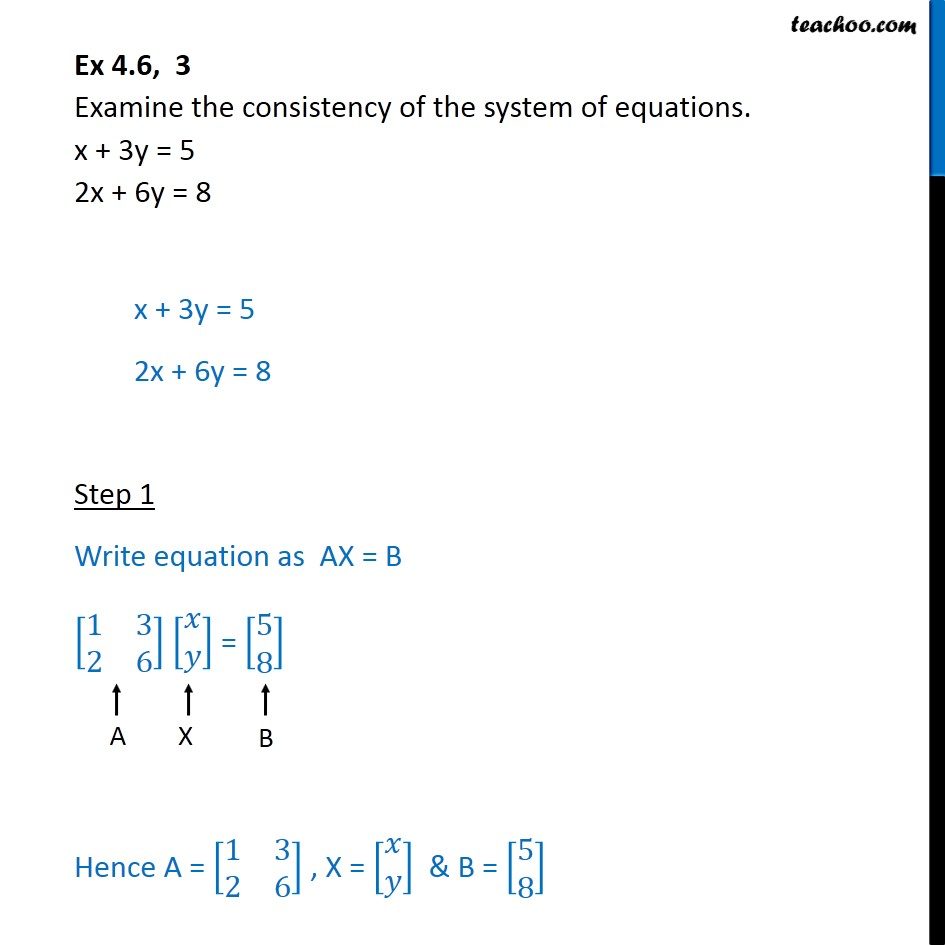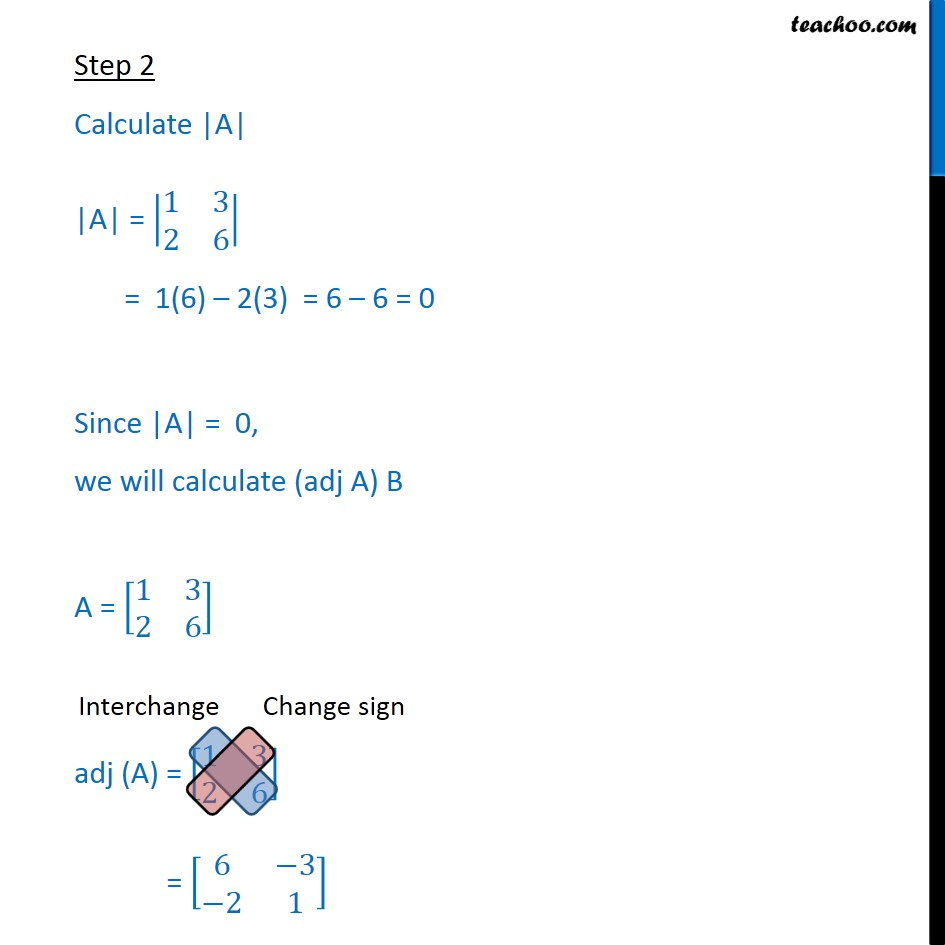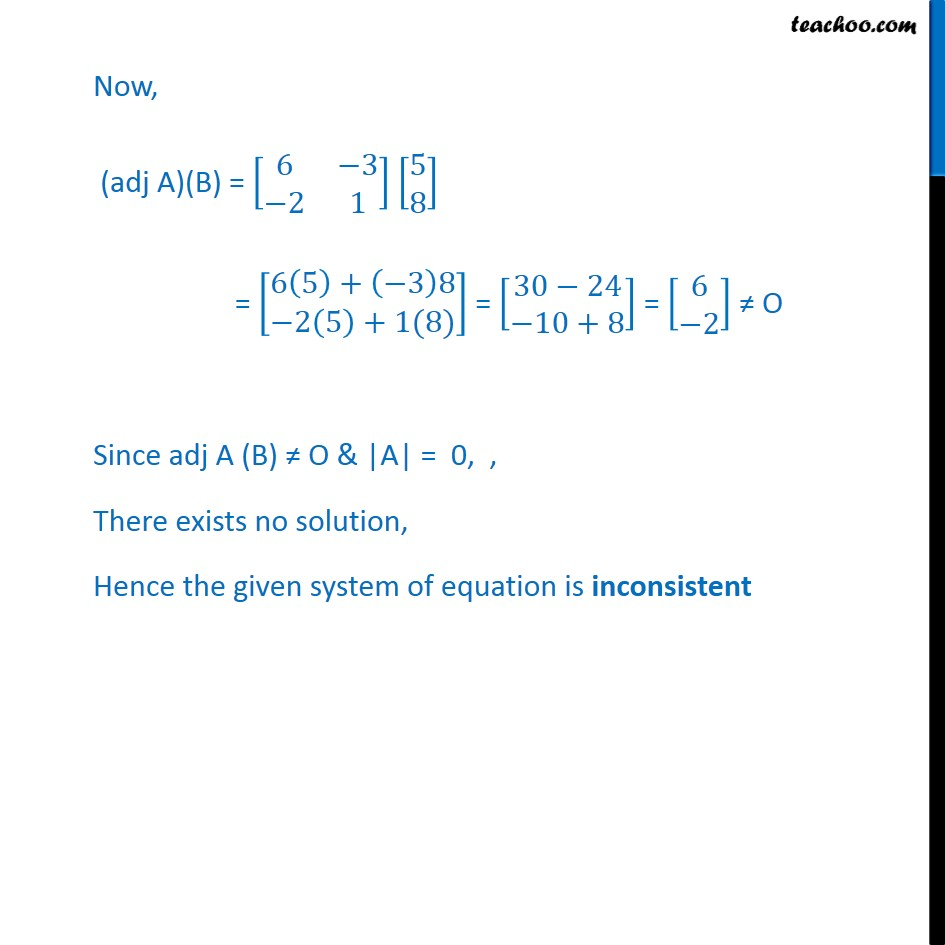1. Chapter 4 Class 12 Determinants
2. Serial order wise
3. Ex 4.6

Transcript

Ex 4.6, 3 Step 2 Calculate |A| |A| = 1 3 2 6 = 1(6) 2(3) = 6 6 = 0 Since |A| = 0, we will calculate (adj A) B A = 1 3 2 6 adj (A) = 1 3 2 6 = 6 3 2 1 Now, (adj A)(B) = 6 3 2 1 5 8 = 6 5 + 3 8 2 5 +1(8) = 30 24 10+8 = 6 2 O Since adj A (B) O & |A| = 0, , There exists no solution, Hence the given system of equation is inconsistent

Ex 4.6